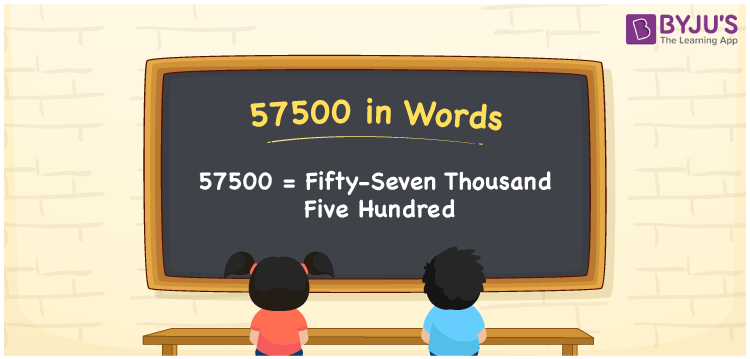# 57500 in Words

57500 in words can be written as Fifty-seven Thousand Five Hundred. The fundamental concepts in Mathematics like counting or count can be learnt efficiently here. If you buy a bike for Rs. 57500, then you can say that “I bought a bike for Fifty-seven Thousand Five Hundred Rupees”. To write numbers in words, the English alphabet is used. The numbers in words concept is explained here in a simple way to improve the conceptual knowledge of students. The 57500 can be read as “Fifty-seven Thousand Five Hundred” in English.

 57500 in words Fifty-seven Thousand Five Hundred Fifty-seven Thousand Five Hundred in Numbers 57500

## 57500 in English Words## How to Write 57500 in Words?

Students can learn about the expanded form and the place value chart of 57500. Five digits are present in the number 57500. With the help of the place value chart given below, students will be able to understand the concepts with ease.

 Ten Thousands Thousands Hundreds Tens Ones 5 7 5 0 0

57500 can be written in expanded form as:

5 x Ten Thousand + 7 x Thousand + 5 × Hundred + 0 × Ten + 0 × One

= 5 x 10000 + 7 x 1000 + 5 × 100 + 0 × 10 + 0 × 1

= 50000 + 7000 + 500

= 57500

= Fifty-seven Thousand Five Hundred

Hence, 57500 in words is written as Fifty-seven Thousand Five Hundred.

57500 is a natural number that precedes 57501 and succeeds 57499.

57500 in words – Fifty-seven Thousand Five Hundred

Is 57500 an odd number? – No

Is 57500 an even number? – Yes

Is 57500 a perfect square number? – No

Is 57500 a perfect cube number? – No

Is 57500 a prime number? – No

Is 57500 a composite number? – Yes

## Frequently Asked Questions on 57500 in Words

Q1

### How to write 57500 in words?

57500 can be written in words as “Fifty-seven Thousand Five Hundred”.
Q2

### How to write Fifty-seven Thousand Five Hundred in numbers?

Fifty-seven Thousand Five Hundred in numbers can be written as 57500.
Q3

### Is 57500 an odd or even number?

57500 is an even number as it is completely divisible by 2.